# References in C++

## References in C++

While creating reference variables, we usually use pointers in C++ but the same task can be done with ‘references’ as well. References work like pointers but are not as powerful as pointers because references are limited in use and have some constraints while using it.

The easiest way to understand references is that if we want to have more than one names of the same memory location then we can use references as shown in the example given below.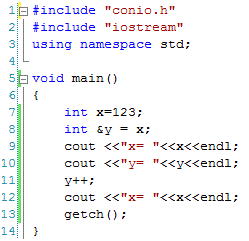In the above code, reference is defined at line 8 (where y is a reference to the memory occupied by the variable x). After this statement, both x and y refers to the same memory location as demonstrated at line 9 and 10. The output of lines 9 and 10 is same (i.e. 123). Even if we change the value of variable y, then the change will be made in variable x as well (as both x and y refers to the same memory). This is demonstrated at lines 11 and 12 above.

The output of the above code is as below.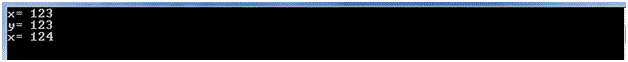Reference Vs. Pointer

1. In contrast to pointer, a reference cannot be null.
2. A reference needs to be initialized at the time of declaration while a pointer can be initialized at the time of declaration or later on.
3. A pointer can refer to different memory locations at different times while a reference can refer to one memory in one function.

References and Functions

References are used in functions if we want to pass the arguments by reference. If used then any change to be made in the value of argument will change the actual argument’s value as well as demonstrated in the following example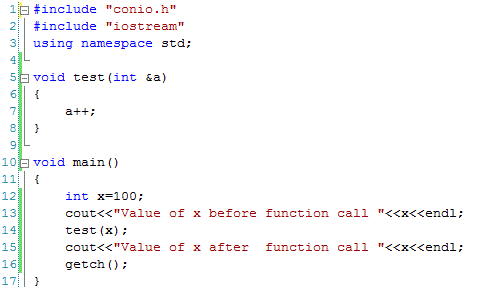At line 5, argument ‘a’ is declared as a reference which will refer to the same memory location as that of variable ‘x’ when the function test is called at line 14. Once the function test is called and we change the value of variable ‘a’ at line 7 then the same value is obtained by variable ‘x’ as well because both ‘x’ and ‘a’ refers to the same memory location.

The output of the above code is as below.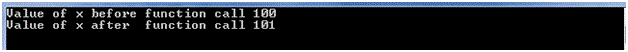Constant Reference

Sometimes we may like that we should not be allowed to change the value of a memory location through reference variable. If this is the case then we can use constant reference. If a reference is declared as constant then compiler will not allow us to change the value as shown in the following example.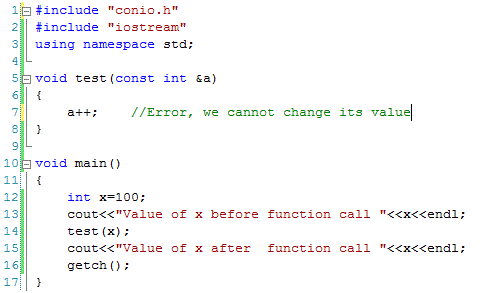As we can see that reference is declared as constant at line 5, so we will not be allowed to change its value otherwise compiler will generate an error message at line 7. This is useful to avoid accidental change of values.